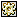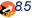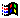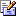Contents Articles Behaviors Books Director News Director Web Sites FAQ Games Mailing Lists News Groups Project Examples Reviews Software Tools Useful Web Sites Utilities Xtras

 Don't miss theseVector Shapes - Create Spiral & Create Spiral Twist

 Compatibilities:This item has not yet been rated

Author: Chunick (website)

These two custom handlers create spiral shapes. When you call one it will return a vertex list which you can set to a vector shape member. The parameters are the number of spirals (integer), width is the spacing between spirals, the direction of the spiral can be an integer or float of almost any value both negative and positive. Experiment to see what happens (hint: 0 turns it into a square spiral and 1 for normal usage) and the constant which is an optional parameter and can generally be almost any value as well.

 on createSpiral (spirals, width, direction, constant)      vertList = []      c1 = 0.585   if the paramCount = 4 then c1 = constant   c2 = c1 * 1.12      int = 90 * pi/180.0   t = 0   spirals = spirals * 4      repeat with a = 1 to spirals     r0 = ((a - 1) + width * t)     t = t + int     r1 = (a + width * t)     x =  r1 * cos(t)     y = r1 * sin(t)     x1 = 0     x2 = 0     y1 = 0     y2 = 0     Case (a mod 4) of       1: -- 1, 90         x1 = -r1 * c2 * direction         x2 = r0 * c1 * direction       2: -- 2, 180         y1 = -r1 * c2 * direction         y2 = r0 * c1 * direction       3: -- 3, 270         x1 = r1 * c2 * direction         x2 = -r0 * c1 * direction       0: -- 4, 360         y1 = r1 * c2 * direction         y2 = -r0 * c1 * direction     end Case          vertList.add([#vertex: point(x, y), #handle1: point(x1,y1), #handle2: point(x2,y2)])        end repeat      return vertList    end on createSpiralTwist (spirals, width, direction, constant)      vertList = []      c = 0.585   if the paramCount = 4 then c = constant      int = 90 * pi/180.0   t = 0   spirals = spirals * 4      repeat with a = 1 to spirals     t = t + int     r = (a + width * t)     x =  r * cos(t)     y = r * sin(t)          x1 = (r * c) * cos(t) * direction     x2 = -x1 * direction     y1 = (r * c) * sin(t) * direction     y2 = -y1 * direction          vertList.add([#vertex: point(x, y), #handle1: point(x1,y1), #handle2: point(x2,y2)])        end repeat      return vertList    end

 Contact MMI 36 South Court Sq Suite 300 Newnan, GA 30263 USASend e-mail